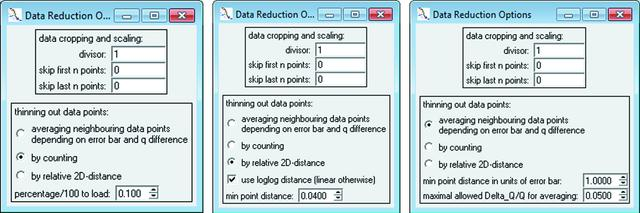disable zoom     view article Figure 1 Data reduction window, providing three different methods for reducing the number of data points. Method one (leftmost figure) skips data points by a count ratio [see equation (2)]. For fast data fitting of 103 data points, a typical fraction of 0.1 is recommended. Method two (middle figure) is a fast method for data with distinctive curve features [see equation (3)]. Method three (right-hand figure) averages data points adaptively according to intensity and q spread [see equation (6)].JOURNAL OFAPPLIEDCRYSTALLOGRAPHY
ISSN: 1600-5767
Volume 48| Part 5| October 2015| Pages 1587-1598